# IB DP Physics: HL复习笔记10.1.2 Gravitational & Electrostatic Field Lines

### Gravitational & Electrostatic Field Lines

#### Gravitational Field Lines

• The direction of a gravitational field is represented by gravitational field lines
• The gravitational field lines around a point mass are radially inwards
• The gravitational field lines of a uniform field, where the field strength is the same at all points, is represented by equally spaced parallel lines
• For example, the fields lines on the Earth’s surface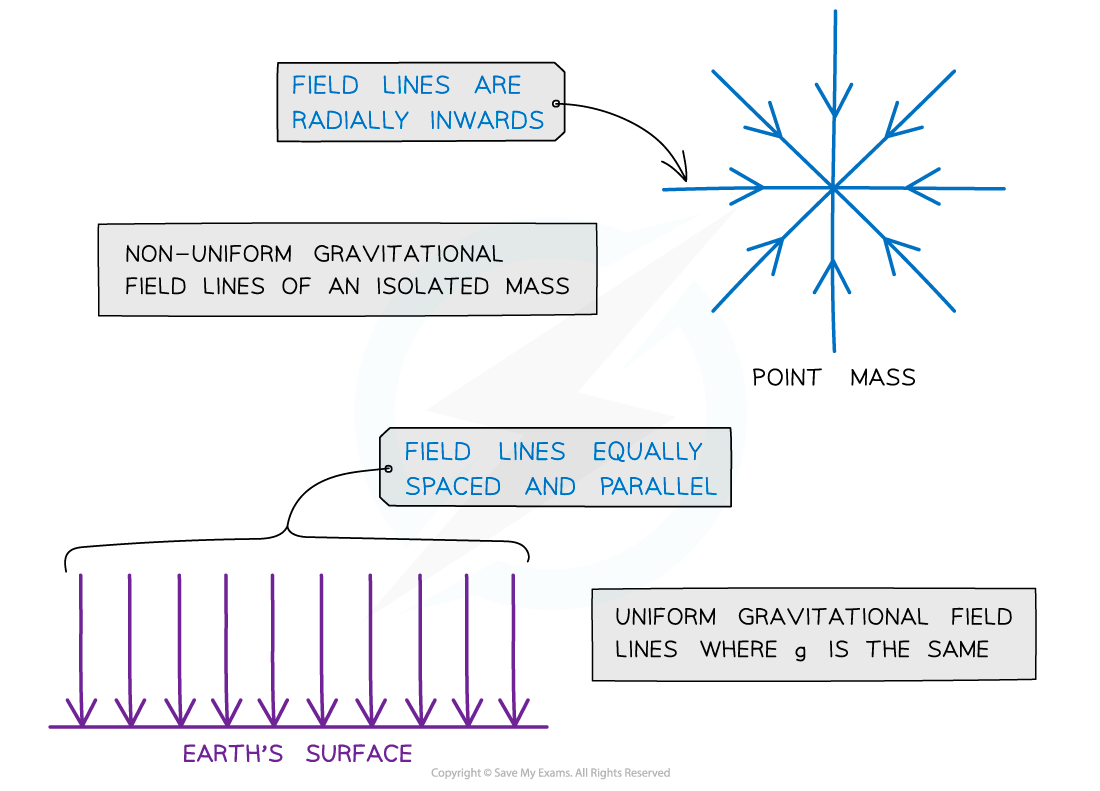Gravitational field lines for a point mass and a uniform gravitational field

• Radial fields are considered non-uniform fields
• The gravitational field strength g is different depending on how far you are from the centre
• Parallel field lines on the Earth’s surface are considered a uniform field
• The gravitational field strength g is the same throughout

#### Point Mass Approximation

• For a point outside a uniform sphere, the mass of the sphere may be considered to be a point mass at its centre
• A uniform sphere is one where its mass is distributed evenly
• The gravitational field lines around a uniform sphere are therefore identical to those around a point mass
• An object can be regarded as point mass when:

A body covers a very large distance as compared to its size, so, to study its motion, its size or dimensions can be neglected

• An example of this is field lines around planets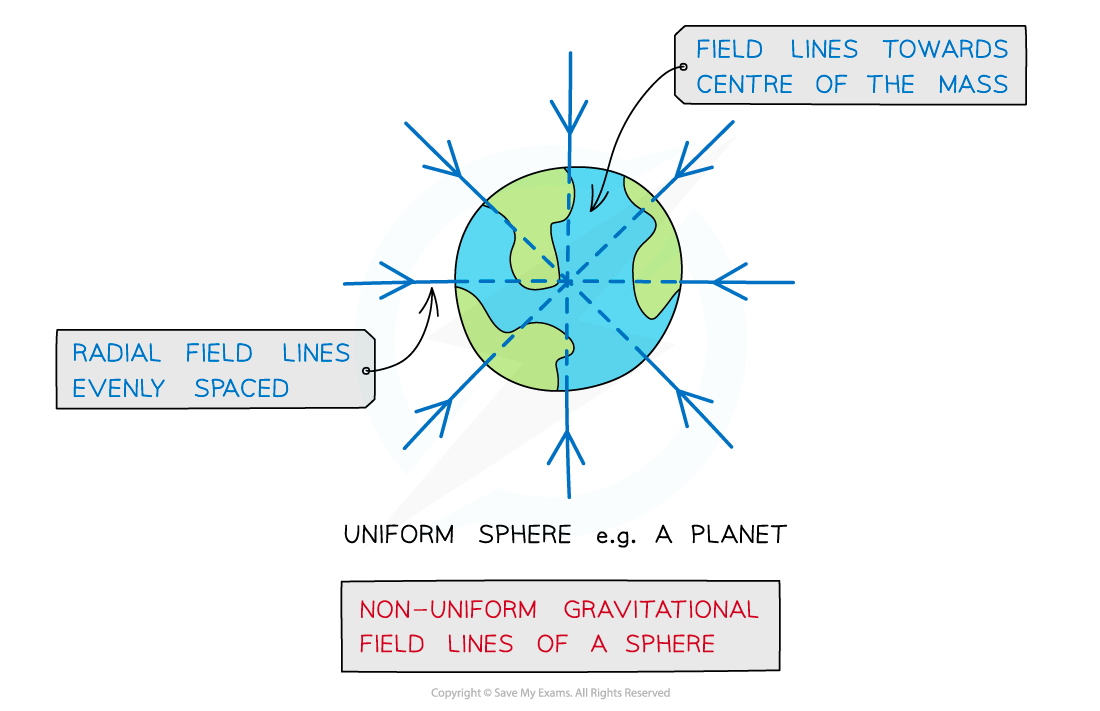Gravitational field lines around a uniform sphere are identical to those on a point mass

• Radial fields are considered non-uniform fields
• So, the gravitational field strength g is different depending on how far an object is from the centre of mass of the sphere

#### Exam Tip

Always label the arrows on the field lines! Gravitational forces are attractive only. Remember:

• For a radial field: it is towards the centre of the sphere or point charge
• For a uniform field: towards the surface of the object e.g. Earth

#### Representing Electric Fields

• The direction of electric fields is represented by electric field lines
• Electric field lines are directed from positive to negative
• Therefore, the field lines must be pointed away from the positive charge and towards the negative charge
• A radial field spreads uniformly to or from the charge in all directions
• e.g. the field around a point charge or sphere
• Around a point charge, the electric field lines are directly radially inwards or outwards:
• If the charge is positive (+), the field lines are radially outwards
• If the charge is negative (-), the field lines are radially inwards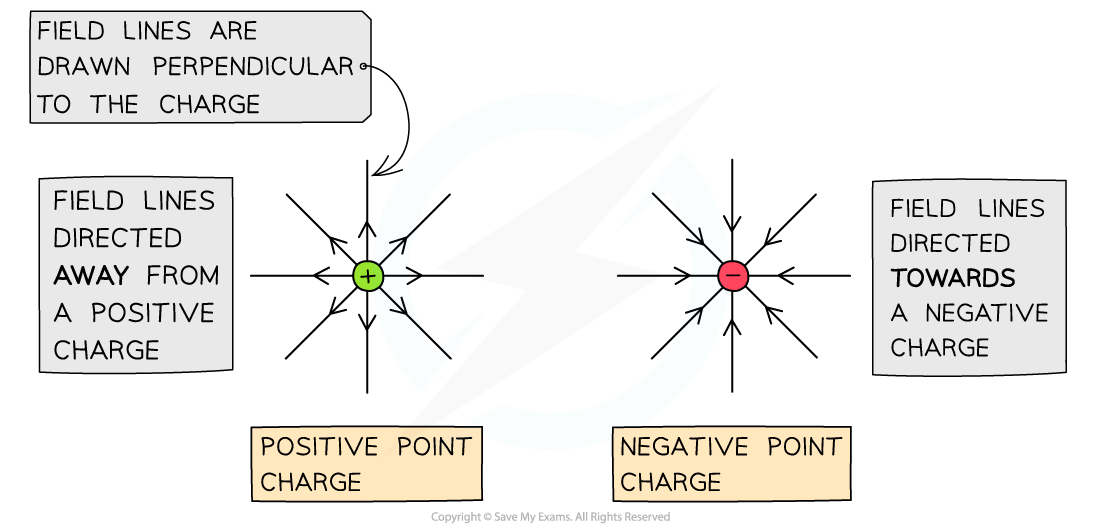Electric field lines point away from a positive charge and point towards a negative charge

• This shares many similarities to radial gravitational field lines around a point mass
• Since gravity is only an attractive force, the field lines will look similar to the negative point charge, whilst electric field lines can be in either direction
• A uniform electric field has the same electric field strength throughout the field
• For example, the field between oppositely charged parallel plates
• This is represented by equally spaced field lines and shares many similarities to uniform gravitational field lines on the surface of a planet
• non-uniform electric field has varying electric field strength throughout
• The strength of an electric field is represented by the spacing of the field lines:
• stronger field is represented by the field lines which are closer together
• weaker field is represented by the field lines which are  further apart
• The electric field lines are directed from the positive to the negative plate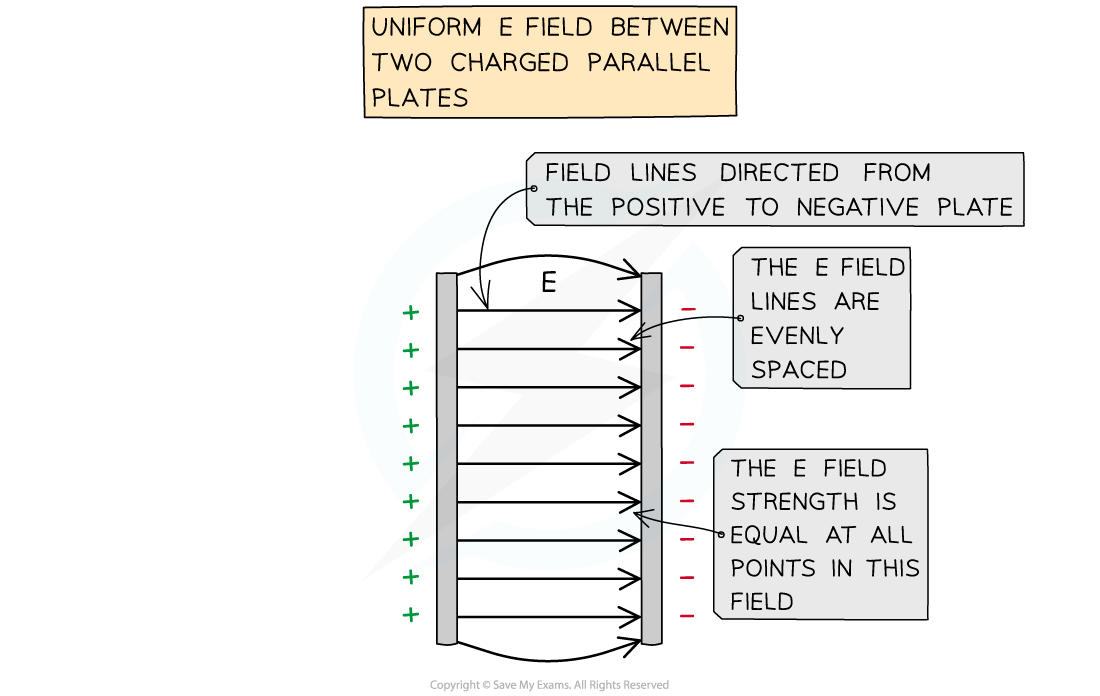The electric field between two parallel plates is directed from the positive to the negative plate. A uniform E field has equally spaced field lines

• A radial field is considered a non-uniform field
• Electric field strength E varies with distance from a charged particle

#### Worked Example

Sketch the electric field lines between the two point charges in the diagram below.Electric field lines around point charges have arrows which point radially outwards for positive charges and radially inwards for negative charges

• Arrows (representing force on a positive test charge) point from the positive charge to the negative charge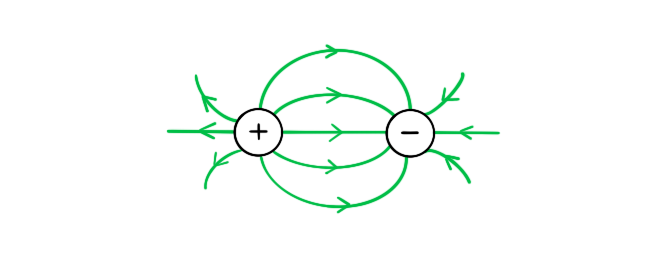#### Exam Tip

Always label the arrows on the field lines! The lines must also touch the surface of the source charge or plates.